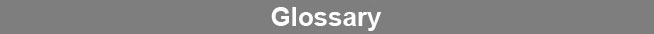Select Page### Analysis of Variance

Analysis of variance (ANOVA) is a statistical test that compares the distribution of two or more sample groups to determine whether one or more of the groups are significantly different from the others. The sample groups must be Gaussian in distribution. In an analysis of variation, the average variance within each of the sample groups is factored out from the variance between each of the sample groups before computing the probability of significant differences between the groups.www.brendan.com